# Introduction

The Microbiome Batch-Effect Correction Suite aims to provide a toolkit for stringent assessment and correction of batch-effects in microbiome data sets. To that end, the package offers wrapper-functions to summarize study-design and data, e.g., PCA, Heatmap and Mosaic-plots, and to estimate the proportion of variance that can be attributed to the batch effect. The `mbecsCorrection()` function acts as a wrapper for various batch effect correcting algorithms (BECA) and in conjunction with the aforementioned tools, it can be used to compare the effectiveness of correction methods on particular sets of data. All functions of this package are accessible on their own or within the preliminary and comparative report pipelines respectively.

## Dependencies {.unnumbered}

The `MBECS` package relies on the following packages to work:

Table: MBECS package dependencies

Package Version Date Repository
phyloseq 1.42.0 2021-11-29 NULL
magrittr 2.0.3 NULL CRAN
cluster 2.1.4 2022-08-19 CRAN
dplyr 1.0.10 NULL CRAN
ggplot2 3.3.6 NULL CRAN
gridExtra 2.3 NULL CRAN
limma 3.54.0 2022-11-01 NULL
lme4 1.1.31 NULL CRAN
lmerTest 3.1.3 NULL CRAN
pheatmap 1.0.12 2018-12-26 CRAN
rmarkdown 2.17 NULL CRAN
ruv 0.9.7.1 2019-08-30 CRAN
sva 3.46.0 NULL NULL
tibble 3.1.8 NULL CRAN
tidyr 1.2.1 NULL CRAN
vegan 2.6.4 NULL CRAN
methods 4.2.1 NULL NULL
stats 4.2.1 NULL NULL
utils 4.2.1 NULL NULL

## Installation {.unnumbered}

`MBECS` should be installed as follows:

``````if (!"BiocManager" %in% rownames(installed.packages()))
install.packages("BiocManager")
BiocManager::install("MBECS")
``````

To install the most current (but not necessarily stable) version, use the repository on GitHub:

``````# Use the devtools package to install from a GitHub repository.
install.packages("devtools")

# This will install the MBECS package from GitHub.
devtools::install_github("rmolbrich/MBECS")
``````

# Workflow

The main application of this package is microbiome data. It is common practice to use the `phyloseq` [@R-phyloseq] package for analyses of this type of data. To that end, the `MBECS` package extends the `phyloseq` class in order to provide its functionality. The user can utilize objects of class `phyloseq` or a `list` object that contains an abundance table as well as meta data. The package contains a dummy data-set of artificially generated data to illustrate this process. To start an analysis, the user requires the `mbecProcessInput()` function.

Load the package via the `library()` function.

``````library(MBECS)
``````

Use the `data()`function to load the provided mockup data-sets at this point.

``````# List object
data(dummy.list)
# Phyloseq object
data(dummy.ps)
# MbecData object
data(dummy.mbec)
``````

## Start from abundance table

For an input that consists of an abundance table and meta-data, both tables require sample names as either row or column names. They need to be passed in a `list` object with the abundance matrix as first element. The `mbecProcessInput()` function will handle the correct orientation and return an object of class `MbecData`.

``````# The dummy-list input object comprises two matrices:
names(dummy.list)
#>  "cnts" "meta"
``````

The optional argument `required.col` may be used to ensure that all covariate columns that should be there are available. For the dummy-data these are “group”, “batch” and “replicate”.

``````mbec.obj <- mbecProcessInput(dummy.list,
required.col = c("group", "batch", "replicate"))
``````

## Start from phyloseq object

The start is the same if the data is already of class `phyloseq`. The `dummy.ps` object contains the same data as `dummy.list`, but it is of class `phyloseq`. Create an `MbecData` object from `phyloseq` input.

The optional argument `required.col` may be used to ensure that all covariate columns that should be there are available. For the dummy-data these are “group”, “batch” and “replicate”.

``````mbec.obj <- mbecProcessInput(dummy.ps,
required.col = c("group", "batch", "replicate"))
``````

## Apply transformations

The most common normalizing transformations in microbiome analysis are total sum scaling (TSS) and centered log-ratio transformation (CLR). Hence, the `MBECS` package offers these two methods. The resulting matrices will be stored in their respective `slots (tss, clr)` in the `MbecData` object, while the original abundance table will remain unchanged.

Use `mbecTransform()` to apply total sum scaling to the data.

``````mbec.obj <- mbecTransform(mbec.obj, method = "tss")
#> Set tss-transformed counts.
``````

Apply centered log-ratio transformation to the data. Due to the sparse nature of compositional microbiome data, the parameter `offset` may be used to add a small offset to the abundance matrix in order to facilitate the CLR transformation.

``````mbec.obj <- mbecTransform(mbec.obj, method = "clr", offset = 0.0001)
``````

## Preliminary report

The function `mbecReportPrelim()` will provide the user with an overview of experimental setup and the significance of the batch effect. To that end it is required to declare the covariates that are related to batch effect and group effect respectively. In addition it provides the option to select the abundance table to use here. The CLR transformed abundances are the default and the function will calculate them if they are not present in the input. Technically, the user can start the analysis at this point because the function incorporates the functionality of the aforementioned processing functions.

The parameter `model.vars` is a character vector with two elements. The first denotes the covariate column that describes the batch effect and the second one should be used for the presumed biological effect of interest, e.g., the group effect in case/control studies. The `type` parameter selects which abundance table is to be used “otu”, “clr”, “tss” .

``````mbecReportPrelim(input.obj=mbec.obj, model.vars=c("batch","group"),
type="clr")
``````

## Run corrections

The package acts as a wrapper for six different batch effect correcting algorithms (BECA).

• Remove Unwanted Variation 3 (`ruv3`)

• This algorithm is implemented in ruv-package by @R-ruv.
• The algorithm requires negative control-features, i.e., features that are known to be unaffected by the batch effect, as well as technical replicates. The algorithm will check for the existence of a replicate column in the covariate data. If the column is not present, the execution stops and a warning message will be displayed. The denominators for negative controls can be supplied via the parameter ‘nc.features’. If they are not supplied, the function will employ a linear model to determine pseudo negative controls that are not significantly affected by the batch effect.
• Batch Mean Centering (`bmc`)

• This algorithm is part of this package.
• For known batch effects, this method takes the batches, i.e., subgroup of samples within a particular batch, and centers them to their mean value.
• ComBat (`bat`)

• Described by Johnson et al. 2007 this method was initially conceived to work with gene expression data and is part of the sva-package by @R-sva.
• This method uses an non-/parametric empirical Bayes framework to correct for known batch effects.
• Remove Batch Effect (`rbe`)

• As part of the limma-package by @R-limma this method was designed to remove BEs from Micro array data.
• The algorithm fits the full-model to the data, i.e., all relevant covariates whose effect should not be removed, and a model that only contains the known batch effects. The difference between these models produces a residual matrix that (presumably) contains only the full-model-effect, e.g., treatment.
• Percentile Normalization (`pn`)

• This method was actually developed specifically to facilitate the integration of microbiome data from different studies/experimental set-ups by @gibbons2018. This problem is similar to the mitigation of BEs, i.e., when collectively analyzing two or more data-sets, every study is effectively a batch on its own (not withstanding the probable BEs within studies).
• The algorithm iterates over the unique batches and converts the relative abundance of control samples into their percentiles. The relative abundance of case-samples within the respective batches is then transformed into percentiles of the associated control-distribution. Basically, the procedure assumes that the control-group is unaffected by any effect of interest, e.g., treatment or sickness, but both groups within a batch are affected by that BE. The switch to percentiles (kinda) flattens the effective difference in count values due to batch - as compared to the other batches. This also introduces the two limiting aspects in percentile normalization. It can only be applied to case/control designs because it requires a reference group. In addition, the transformation into percentiles removes information from the data.
• The ‘mbecRunCorrections’ wrapper will automatically select Total Sum Scaled values for percentile normalization because that is what it is supposed to be used on. The function `mbecCorrections` can be used to manually select untransformed or centered log ratio transformed abundances.
• Support Vector Decomposition (`svd`)

• Successfully applied to micro-array data by @nielsen2002.
• Basically perform matrix factorization and compute singular eigen-vectors (SEV). Assume that the first SEV captures the batch-effect and remove this effect from the data.

The user has the choice between two functions `mbecCorrection()` and `mbecRunCorrections()`, the latter one acts as a wrapper that can apply multiple correction methods in a single run. If the input to `mbecRunCorrections()` is missing CLR and TSS transformed abundance matrices, they will be created with default settings, i.e., offsets for zero values will be set to 1/#features.

The function `mbecCorrection()` will apply a single correction algorithm selected by the parameter `method` and return an object that contains the resulting corrected abundance matrix in its `cor slot` with the respective name.

``````mbec.obj <- mbecCorrection(mbec.obj, model.vars=c("batch","group"),
method = "bat", type = "clr")
#> Applying ComBat (sva) for batch-correction.
#> Found2batches
#> Adjusting for1covariate(s) or covariate level(s)
#> Standardizing Data across genes
#> Fitting L/S model and finding priors
``````

The function `mbecRunCorrections()` will apply all correction algorithms selected by the parameter `method` and return an object that contains all respective corrected abundance matrices in the `cor` slot. In this example there will be three in total, named like the methods that created them.

``````mbec.obj <- mbecRunCorrections(mbec.obj, model.vars=c("batch","group"),
method=c("ruv3","rbe","bmc","pn","svd"),
type = "clr")
#> No negative control features provided.
#>                 Using pseudo-negative controls.
#> Applying Remove Unwanted Variantion v3 (RUV-III).
#> Applying 'removeBatchEffect' (limma) for batch-correction.
#> Applying Batch Mean-Centering (BMC) for batch-correction.
#> Applying Percentile Normalization (PN).
#> Warning in mbecPN(input.obj, model.vars, type = eval(type)): Abundances contain
#> zero values. Adding small uniform offset.
#> Group A is considered control group, i.e., reference
#>           for normalization procedure. To change reference please 'relevel()'
#>           grouping factor accordingly.
#> Applying Singular Value Decomposition (SVD) for batch-correction.
``````

## Post report

The `mbecReportPost()` function will provide the user with a comparative report that shows how the chosen batch effect correction algorithms changed the data-set compared to the initial values.

The parameter `model.vars` is a character vector with two elements. The first denotes the covariate column that describes the batch effect and the second one should be used for the presumed biological effect of interest, e.g., the group effect in case/control studies. The `type` parameter selects which abundance table is to be used “otu”, “clr”, “tss” .

``````mbecReportPost(input.obj=mbec.obj, model.vars=c("batch","group"),
type="clr")
``````

## Retrieve corrrected data

Because the `MbecData` class extends the `phyloseq` class, all functions from `phyloseq` can be used as well. They do however only apply to the `otu_table` slot and will return an object of class `phyloseq`, i.e., any transformations or corrections will be lost. To retrieve an object of class `phyloseq` that contains the `otu_table` of corrected counts, for downstream analyses, the user can employ the `mbecGetPhyloseq()` function. As before, the arguments `type` and `label` are used to specify which abundance table should be used in the returned object.

To retrieve the CLR transformed counts, set `type` accordingly.

``````ps.clr <- mbecGetPhyloseq(mbec.obj, type="clr")
``````

If the batch-mean-centering corrected counts show the best results, select “cor” as `type` and set the `label` to “bmc”.

``````ps.bmc <- mbecGetPhyloseq(mbec.obj, type="cor", label="bmc")
``````

# Use single functions

## Exploratory Functions

### Relative Log-Expression

Relative Log-Expression plots can be created with the `mbecRLE()` function.

Produce RLE-plot on CLR transformed values. The `return.data` parameter can be set to TRUE to retrieve the data for inspection or use in your own plotting function.

``````rle.plot <- mbecRLE(input.obj=mbec.obj, model.vars = c("batch","group"),
type="clr",return.data = FALSE)
#> Calculating RLE for group: A
#> Calculating RLE for group: B

# Take a look.
plot(rle.plot)
``````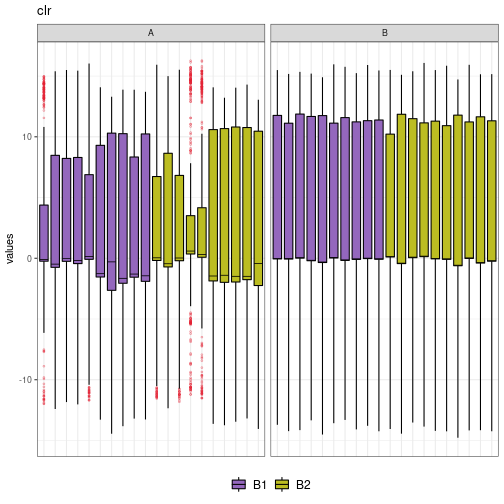### Principal Components Analysis

PCA plots can be created with the `mbecPCA()` function.

Produce PCA-plot on CLR transformed values. The principal components can be selected with the `pca.axes` parameter. The vector of length two works like this c(x-axis, y-axis). The return data parameter can be set to TRUE to retrieve the data for inspection or use in your own plotting function.

``````pca.plot <- mbecPCA(input.obj=mbec.obj, model.vars = "group", type="clr",
pca.axes=c(1,2), return.data = FALSE)
``````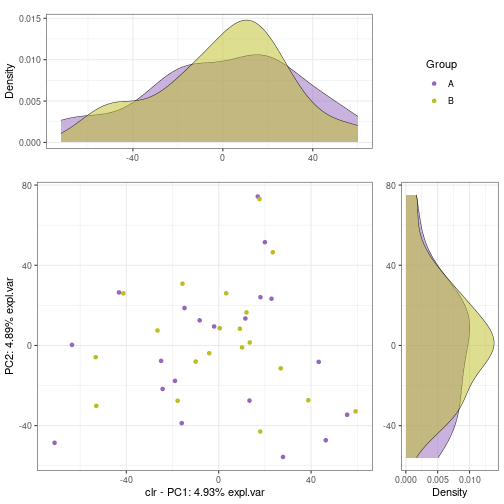Plot with two grouping variables, determining color and shape of the output.

``````pca.plot <- mbecPCA(input.obj=mbec.obj, model.vars = c("batch","group"),
type="clr", pca.axes=c(1,2), return.data = FALSE)
``````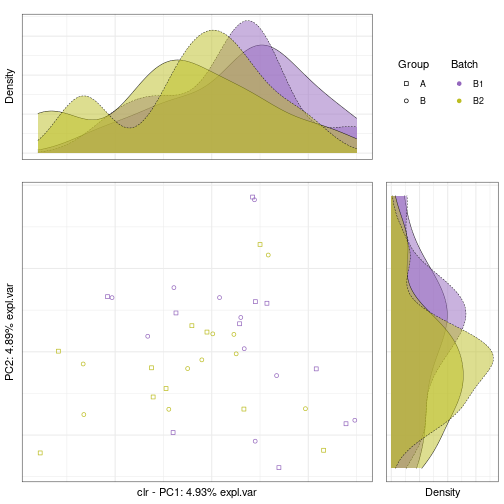### Box Plot

Box plots of the most variable features can be create with the `mbecBox()` function.

Produce Box-plots of the most variable features on CLR transformed values. The `method` parameter determines if “ALL” or only the “TOP” n features are plotted. The `n` parameter selects the number of features to plot. The function will return a `list` of plot objects to be used.

``````box.plot <- mbecBox(input.obj=mbec.obj, method = "TOP", n = 2,
model.var = "batch", type="clr", return.data = FALSE)

# Take a look.
plot(box.plot\$OTU109)
``````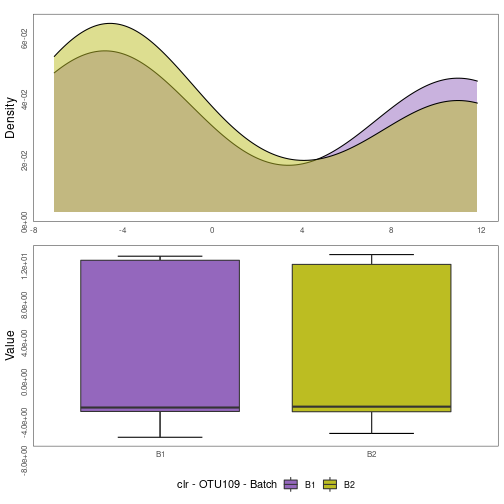Setting `method` to a vector of feature names will select exactly these features for plotting.

``````box.plot <- mbecBox(input.obj=mbec.obj, method = c("OTU1","OTU2"),
model.var = "batch", type="clr", return.data = FALSE)
#> 'Method' parameter contains multiple elements -
#>             using to select features.

# Take a look.
plot(box.plot\$OTU2)
``````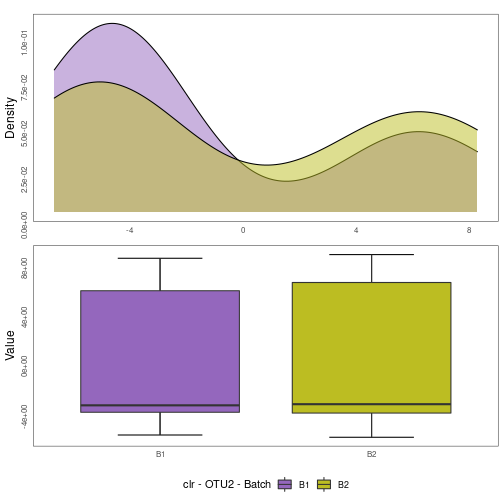### Heatmap

Pheatmap is used to produce heat-maps of the most variable features with the `mbecHeat()` function.

Produce a heatmap of the most variable features on CLR transformed values. The `method` parameter determines if “ALL” or only the “TOP” n features are plotted. The `n` parameter selects the number of features to plot. The parameters `center` and `scale` can be used to de-/activate centering and scaling prior to plotting. The `model.vars` parameter denotes all covariates of interest.

``````heat.plot <- mbecHeat(input.obj=mbec.obj, method = "TOP", n = 10,
model.vars = c("batch","group"), center = TRUE,
scale = TRUE, type="clr", return.data = FALSE)
``````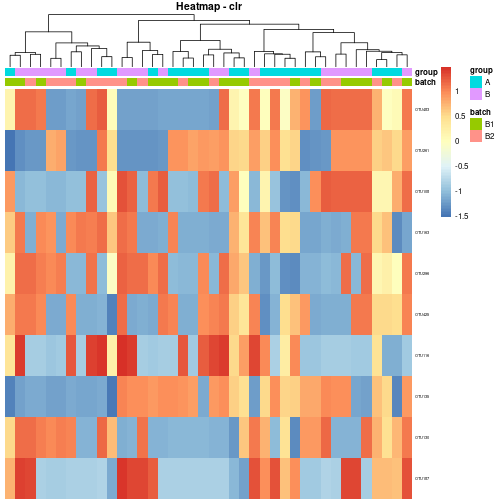### Mosaic

A mosaic plot of the distribution of samples over two covariates of interest can be produced with the `mbecMosaic()` function.

``````mosaic.plot <- mbecMosaic(input.obj = mbec.obj,
model.vars = c("batch","group"),
return.data = FALSE)
``````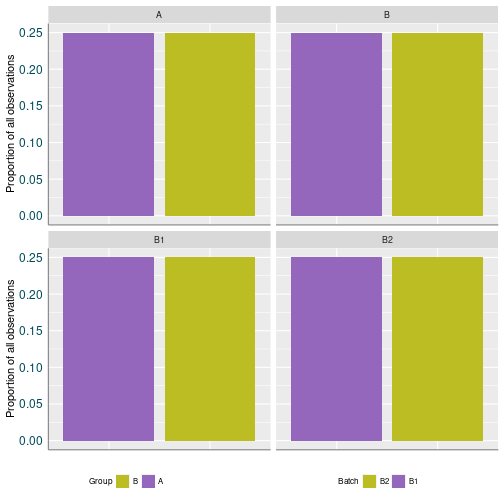## Analysis of Variance

The functions aim to determine the amount of variance that can be attributed to selected covariates of interest and visualize the results.

### Linear Model

Use the function `mbecModelVariance()` with parameter `method` set to “lm” to fit a linear model of the form `y ~ group + batch` to every feature. Covariates of interest can be selected with the `model.vars` parameter and the function will construct a model-formula. The parameters `type` and `label` can be used to select the desired abundance matrix to work on This defaults to CLR transformed values.

Plot the resulting data with the `mbecVarianceStatsPlot()` function and show the proportion of variance with regards to the covariates in a box-plot.

``````lm.variance <- mbecModelVariance(input.obj=mbec.obj,
model.vars = c("batch", "group"),
method="lm",type="cor", label="svd")

# Produce plot from data.
lm.plot <- mbecVarianceStatsPlot(lm.variance)

# Take a look.
plot(lm.plot)
``````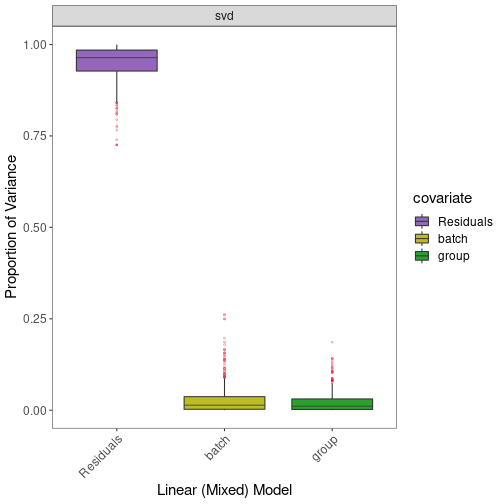### Linear Mixed Model

Use the function `mbecModelVariance()` with parameter `method` set to “lmm” to fit a linear mixed model of the form `y ~ group + (1|batch)` to every feature. Covariates of interest can be selected with the `model.vars` parameter and the function will construct a model-formula. The parameters `type` and `label` can be used to select the desired abundance matrix to work on This defaults to CLR transformed values.

Plot the resulting data with the `mbecVarianceStatsPlot()` function and show the proportion of variance with regards to the covariates in a box-plot.

``````lmm.variance <- mbecModelVariance(input.obj=mbec.obj,
model.vars = c("batch","group"),
method="lmm",
type="cor", label="ruv3")

# Produce plot from data.
lmm.plot <- mbecVarianceStatsPlot(lmm.variance)

# Take a look.
plot(lmm.plot)
``````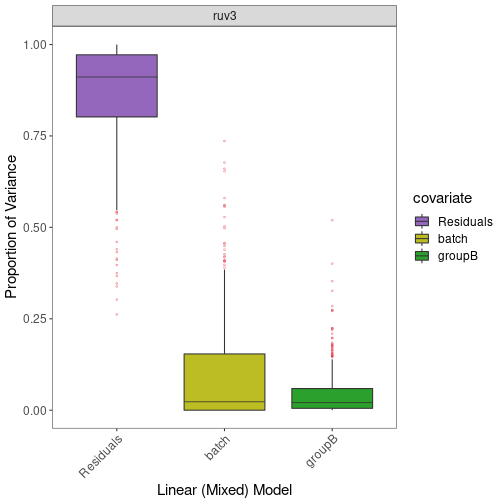### Redundancy Analysis

Use the function `mbecModelVariance()` with parameter `method` set to “rda” to calculate the percentage of variance that can be attributed to the covariates of interest with partial Redundancy Analysis. Covariates of interest can be selected with the `model.vars` parameter. The parameters `type` and `label` can be used to select the desired abundance matrix to work on This defaults to CLR transformed values.

Plot the resulting data with the `mbecRDAStatsPlot()` function and show the percentage of variance with regards to the covariates in a bar-plot.

``````rda.variance <- mbecModelVariance(input.obj=mbec.obj,
model.vars = c("batch", "group"),
method="rda",type="cor", label="bat")

# Produce plot from data.
rda.plot <- mbecRDAStatsPlot(rda.variance)

# Take a look.
plot(rda.plot)
``````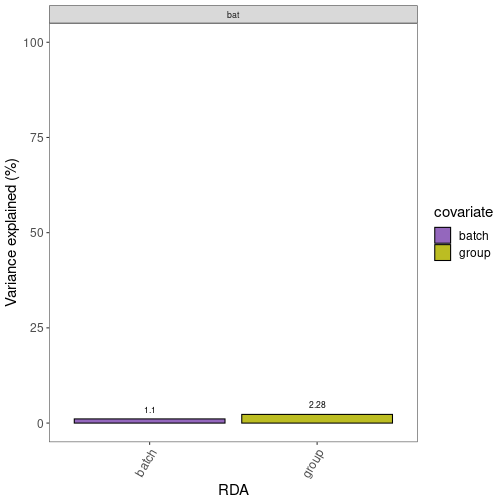### Principal Variance Components Analysis

Use the function `mbecModelVariance()` with parameter `method` set to “pvca” to calculate the percentage of variance that can be attributed to the covariates of interest using Principal Variance Components Analysis. Covariates of interest can be selected with the `model.vars` parameter. The parameters `type` and `label` can be used to select the desired abundance matrix to work on This defaults to CLR transformed values.

Plot the resulting data with the `mbecPVCAStatsPlot()` function and show the percentage of variance with regards to the covariates in a bar-plot.

``````pvca.variance <- mbecModelVariance(input.obj=mbec.obj,
model.vars = c("batch", "group"),
method="pvca",type="cor", label="rbe")

# Produce plot from data.
pvca.plot <- mbecPVCAStatsPlot(pvca.variance)

# Take a look.
plot(pvca.plot)
``````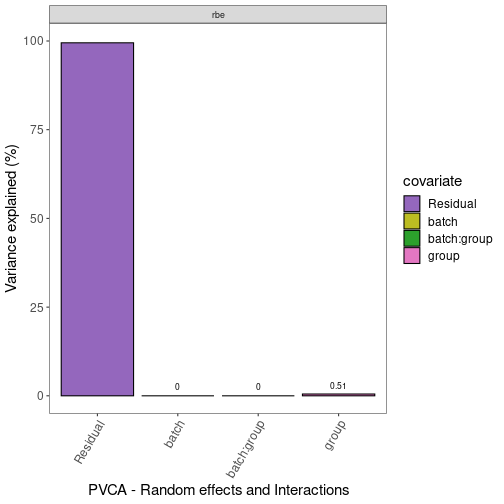### Silhouette Coefficient

Evaluate how good the samples fit to the clustering that is implied by the covariates of interest.

Use the function `mbecModelVariance()` with parameter `method` set to “s.coef” to evaluate the clustering that is implied by the covariates of interest with the Silhouette Coefficient. Covariates of interest can be selected with the `model.vars` parameter. The parameters `type` and `label` can be used to select the desired abundance matrix to work on This defaults to CLR transformed values.

Plot the resulting data with the `mbecSCOEFStatsPlot()` function and show the silhouette coefficients in a dot-plot.

``````sil.coefficient <- mbecModelVariance(input.obj=mbec.obj,
model.vars = c("batch", "group"),
method="s.coef",type="cor", label="bmc")

# Produce plot from data.
scoef.plot <- mbecSCOEFStatsPlot(sil.coefficient)

# Take a look.
plot(scoef.plot)
``````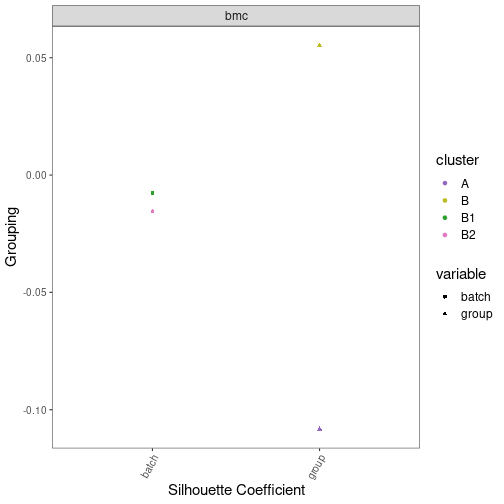# Session

``````#> R version 4.2.1 (2022-06-23)
#> Platform: x86_64-pc-linux-gnu (64-bit)
#> Running under: Ubuntu 20.04.5 LTS
#>
#> Matrix products: default
#> BLAS:   /home/biocbuild/bbs-3.16-bioc/R/lib/libRblas.so
#> LAPACK: /home/biocbuild/bbs-3.16-bioc/R/lib/libRlapack.so
#>
#> attached base packages:
#>  stats     graphics  grDevices utils     datasets  methods   base
#>
#> other attached packages:
#>  MBECS_1.2.0
#>
#> loaded via a namespace (and not attached):
#>    nlme_3.1-160           bitops_1.0-7           matrixStats_0.62.0
#>    phyloseq_1.42.0        bit64_4.0.5            RColorBrewer_1.1-3
#>    httr_1.4.4             GenomeInfoDb_1.34.0    tools_4.2.1
#>   utf8_1.2.2             R6_2.5.1               vegan_2.6-4
#>   DBI_1.1.3              BiocGenerics_0.44.0    mgcv_1.8-41
#>   colorspace_2.0-3       permute_0.9-7          rhdf5filters_1.10.0
#>   gridExtra_2.3          bit_4.0.4              compiler_4.2.1
#>   cli_3.4.1              Biobase_2.58.0         labeling_0.4.2
#>   scales_1.2.1           genefilter_1.80.0      digest_0.6.30
#>   stringr_1.4.1          minqa_1.2.5            XVector_0.38.0
#>   pkgconfig_2.0.3        lme4_1.1-31            fastmap_1.1.0
#>   ruv_0.9.7.1            limma_3.54.0           highr_0.9
#>   rlang_1.0.6            RSQLite_2.2.18         generics_0.1.3
#>   farver_2.1.1           jsonlite_1.8.3         BiocParallel_1.32.0
#>   dplyr_1.0.10           RCurl_1.98-1.9         magrittr_2.0.3
#>   GenomeInfoDbData_1.2.9 biomformat_1.26.0      Matrix_1.5-1
#>   Rcpp_1.0.9             munsell_0.5.0          S4Vectors_0.36.0
#>   Rhdf5lib_1.20.0        fansi_1.0.3            ape_5.6-2
#>   lifecycle_1.0.3        stringi_1.7.8          edgeR_3.40.0
#>   MASS_7.3-58.1          zlibbioc_1.44.0        rhdf5_2.42.0
#>   plyr_1.8.7             grid_4.2.1             blob_1.2.3
#>   parallel_4.2.1         crayon_1.5.2           lattice_0.20-45
#>   Biostrings_2.66.0      splines_4.2.1          multtest_2.54.0
#>   annotate_1.76.0        KEGGREST_1.38.0        locfit_1.5-9.6
#>   knitr_1.40             pillar_1.8.1           igraph_1.3.5
#>   boot_1.3-28            reshape2_1.4.4         codetools_0.2-18
#>   stats4_4.2.1           XML_3.99-0.12          glue_1.6.2
#>   evaluate_0.17          data.table_1.14.4      nloptr_2.0.3
#>   vctrs_0.5.0            png_0.1-7              foreach_1.5.2
#>   gtable_0.3.1           purrr_0.3.5            tidyr_1.2.1
#>   assertthat_0.2.1       cachem_1.0.6           ggplot2_3.3.6
#>   xfun_0.34              xtable_1.8-4           survival_3.4-0
#>  tibble_3.1.8           pheatmap_1.0.12        iterators_1.0.14
#>  AnnotationDbi_1.60.0   memoise_2.0.1          IRanges_2.32.0
#>  cluster_2.1.4          sva_3.46.0             ellipsis_0.3.2
``````